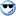CodeGuru Home VC++ / MFC / C++ .NET / C# Visual Basic VB Forums Developer.com

# Thread: calloc-ating memory for 2d array how to?

1. ##calloc-ating memory for 2d array how to?

Is this a/the right way to allocate memory for a 2D array of integer elements with 'num_rows' rows and 'num_columns' columns using double pointer and calloc()?

Code:
```
int **ppi;
int num_rows;
int num_columns;

//(step 1) allocate memory for array of elements of column

ppi = (int** )calloc(num_rows, sizeof(int* ));

//(step 2) allocate memory for array of elements of each row

for( int i = 0; i < num_rows; i++)
*(ppi + i) = (int* )calloc(num_columns, sizeof(int));
//*(ppi + i) = (int* )calloc(1 ???, sizeof(int));```
And is deallocation, respectively done as follows:

Code:
```for(int i = 0; i < num_rows; i++)
free(*(ppi + i));
free(ppi);```
Thanks a lot.

regards,

vpvReply With Quote

2. RFKJunior MemberJoin Date
Dec 2002
Posts
16
Looks pretty good. You start from the double pointer, allocate an array of pointers, and then allocate an array for each allocated pointer. You do rows then columns, which is more efficient than the columns then rows since C is row major, and you use pointer arithmetic instead of operator[] which is more efficient in general. So it seems like a reasonably efficent, and correct implementation. Especially since you have all your *'s in the right place.

and the deallocation looks good too.Reply With Quote

3.Elite Member Power PosterJoin Date
Apr 1999
Posts
27,449

## Re: calloc-ating memory for 2d array how to?

Originally posted by Arnold Layne
Is this a/the right way to allocate memory for a 2D array of integer elements with 'num_rows' rows and 'num_columns' columns using double pointer and calloc()?
Yes, your code looks correct. However, for POD (plain old data types), there is a better solution.

All you need are two allocation calls. One call allocates the pointers (which you did in your code), and another to allocate the entire memory pool. Then you point the allocated pointers to the right places in the memory.

Then when you free() you just need two calls. One call to free the pool of memory, and the second free to deallocate the pointers. Veteran 'C' programmers know this trick, and it enhances the speed of a program greatly, since calls to calloc (or malloc) can be time-consuming. If you have 1000 x 1000 matrix, that is a 1,000 calls to calloc, as opposed to doing only 2 calls to calloc.

Here is the way that I have done this:
Code:
```#include <stdlib.h>

int main()
{
int **ppi;
int num_rows = 1000;
int num_columns = 1000;
int *pool;
int *curPtr;
//(step 1) allocate memory for array of elements of column

ppi = (int** )calloc(num_rows, sizeof(int* ));

//(step 2) allocate memory for array of elements of each row
pool = (int *)calloc( num_columns * num_rows, sizeof(int));

// Now point the pointers in the right place
curPtr = pool;
for( int i = 0; i < num_rows; i++)
{
*(ppi + i) = curPtr;
curPtr += num_columns;
}

ppi = 10;
ppi = 20;

free(*ppi);
free(ppi);
}```
Regards,

Paul McKenzieReply With Quote

4. ##Thanks both of you, guys. You've been of help. I just wasn't very sure for my 2nd step if it should be

Code:
`	*(ppi + i) = (int* )calloc(num_columns, sizeof(int));`
or

Code:
`	*(ppi + i) = (int* )calloc(     1      , sizeof(int));`
But after all you think the 1st call seems correct, right? I only hope you're right.

However, for POD (plain old data types), there is a better solution.
Thanks a bunch for this, Paul! It's extremely valuable trick! I haven't thought of it before, and I've never actually given much thought to the fact that sometimes calloc/malloc are called several hundred times which should cause a great lag after allsincerely,

vpvReply With Quote

5.Member Power PosterJoin Date
May 2000
Location
KY, USA
Posts
18,652
Just for the sake of completeness....the C++ way of dealing with 2d-arrays can be found here...Reply With Quote

####Posting Permissions

• You may not post new threads
• You may not post replies
• You may not post attachments
• You may not edit your posts
•